Courses

# Test: Electrical And Electronic Measurements- 4

## 10 Questions MCQ Test Mock Test Series for Electrical Engineering (EE) GATE 2020 | Test: Electrical And Electronic Measurements- 4

Description
This mock test of Test: Electrical And Electronic Measurements- 4 for Electrical Engineering (EE) helps you for every Electrical Engineering (EE) entrance exam. This contains 10 Multiple Choice Questions for Electrical Engineering (EE) Test: Electrical And Electronic Measurements- 4 (mcq) to study with solutions a complete question bank. The solved questions answers in this Test: Electrical And Electronic Measurements- 4 quiz give you a good mix of easy questions and tough questions. Electrical Engineering (EE) students definitely take this Test: Electrical And Electronic Measurements- 4 exercise for a better result in the exam. You can find other Test: Electrical And Electronic Measurements- 4 extra questions, long questions & short questions for Electrical Engineering (EE) on EduRev as well by searching above.
QUESTION: 1

### A 200 V, 5 A d.c. energy meter is tested at its marked ratings. The resistance of the pressure circuit is 8000 Ω and that of current coil is 0.1 Ω. The power consumed when testing the meter with phantom loading with current circuit excited by a 6 V battery is?

Solution:

Power consumed by current coil = VI = 6 × 5 = 30W
Power consumed by pressure coil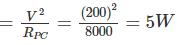Total power = 30 + 5 = 35 W

*Answer can only contain numeric values
QUESTION: 2

### The meter constant of a single phase 240V induction watt-hour meter is 500 revolutions per kWh. The sped of the meter disc for a current of 15A at 0.6 P.f lagging will be ------ (in rpm)

Solution:

P = VI cosϕ = 240 × 15 × 0.6 = 2160 W = 2.16 KW
Meter constant = 500 revolutions/kwh
⇒ For 2.16 kW = 1080 revolutions/hour
= 18 revolution/minute
∴ Speed of disc = 18 rpm

QUESTION: 3

### The braking torque provided by a permanent magnet in a single phase energy meter is proportional to the i) square of the flux of the permanent magnet ii) speed of the meter iii) distance of he permanent magnet from the center of the revolving disc Q. Which of the above statements are correct.

Solution:

The braking torque is provided by the interaction of the eddy current and the field of the permanent magnet. this torque is directly proportional to the product of square of flux of the magnet, magnitude of eddy current and the effective radius R from the axis of the disc. braking torque is directly proportional to speed of rotation.

QUESTION: 4

Which one of the following defects is responsible for creeping in an induction type energy meter?

Solution:

In some meters continuous rotation is obtained even when there is no current passing through the current coil and only pressure coil is energized. This is called creeping. The major cause for creeping is overcompensation for friction.

*Answer can only contain numeric values
QUESTION: 5

A 230 V, single phase watt hour meter has a constant load of 6 A passing through it for 8 hours at unity power factor. If the meter disc makes 2400 revolutions during this period. What is the power factor of the load if the number of revolutions made by the meter are 1800 when operating at 230 V and 8 A for 6 hours?

Solution:

Energy supplied = VI cos ϕ × t × 10-3
= 230 × 6 × 8 × 10-3 = 11.04 kwh
Meter constant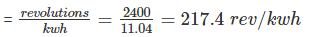Energy consumed when the meter takes 1800 revolutions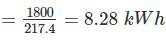Now energy consumed = VI cos ϕ × t × 10-3
8.28 = 230 × cos ϕ × 8 × 6 × 10-3
⇒ cos ϕ = 0.75

*Answer can only contain numeric values
QUESTION: 6

A correctly adjusted single phase, 200 V induction watt meter has a meter constant of 600 revolutions per kWh. Calculate the error introduced at unity PF. (in %), for a current of 8 A at a power factor of 0.8 lagging if the lag adjusted is altered so that the phase angle between voltage coil flux and applied the voltage of 76°.

Solution:

Energy consumed in one minuit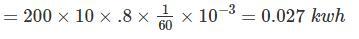= 0.027 × 600 = 16 RPM
Speed of disc = 16 RPM
Steady speed N = KVI sin (Δ – ϕ)
N = KVI sin (90° - ϕ) = KVI cos ϕ
∴ Error introduce because of incorrect lag adjustment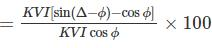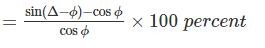We have Δ = 76°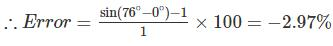QUESTION: 7

The constant for a three phase, 3 element integrating wattmeter is 0.12 revolution of disc per kWh. If the meter is normally used with a potential transformer of ratio 22,000/110V and a current transformer of ratio 500/5A; find the error expressed as a percentage of the correct reading from the following test figures for the instrument only
Line voltage = 100 V; current = 5.25 A; power factor = 1
Time to complete 40 revolutions = 61s.

Solution:

Actual energy consumed during the test period = √3 × ratio of P.T. × ratio of C.T. × VsIs cosϕ × t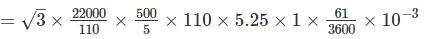= 338.97 kWh
Energy recorded by meter during the test period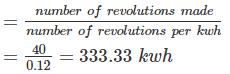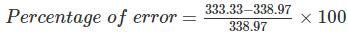= −1.66% = 1.66% low

QUESTION: 8

A single phase energy meter operating on 220 V and 8 A for half day i.e. 12 hours makes 3200 revolutions. If the meter constant = 600 revolutions/kwh, then the power factor of the load will be

Solution:

Actual energy consumed = VI cos ϕ × t × 10-3 kwh where t is in hours
Energy recoded = 3200/600 = 5.33kwh
⇒ 220 × 8 × 12 × cos ϕ × 10-3 = 5.33
Cos ϕ = 0.2525

*Answer can only contain numeric values
QUESTION: 9

A 220 V, single phase, watt hour meter has a constant load of 4 A passing through it for 6 hour at unity power factor. The meter disc makes 2376 revaluation during this period. If the number of revolution made by the meter are 1542 when operating at 220 V and 6 A for 4 hours, then power factor of the load would be

Solution: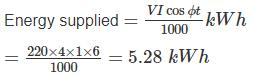Energy meter constant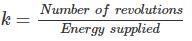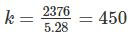Energy consumed when the meter make 1542 revaluations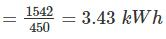Now energy consumed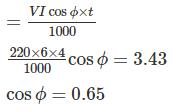QUESTION: 10

A 220V, 10 A dc energy meter is tested for its name plate ratings. Resistance of the pressure coil circuit is 8000 Ω and that of current coil itself is 0.12 Ω calculate the energy consumed when testing for a period of 1 hour with phantom loading with the current coil circuit excited by a separated 9 V battery.

Solution: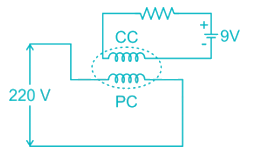Power consumed in the pressure coil circuit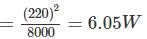Power consumed in the current coil circuit = 9 × 10 = 90 W
Total energy consumed with direct measure = 96.05 W
Total energy consumed during 1 hour with direct measurement = 96.05 × 1 = 96.05 Wh